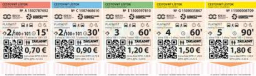# Probability 64764

Petra wrote natural numbers from 1 to 20 on 20 tickets. Milady had one ticket pulled out. What is the probability that Milada will pull out a ticket with a number divisible by three?

p =  0.3

### Step-by-step explanation:Did you find an error or inaccuracy? Feel free to write us. Thank you!

Tips for related online calculators
Need help calculating sum, simplifying, or multiplying fractions? Try our fraction calculator.
Would you like to compute the count of combinations?